}

# Forgery of Physics Newton

1988/02/01 Martinez Lizarduikoa, Alfontso Iturria: Elhuyar aldizkaria

On the occasion of this year's celebration of the 300th anniversary of the publication by the sage Newton of the famous book "Principia Mathematica", I have been asked to refer to the philosophical aspect of Newton's theory. And that's what I'm going to do. I will explain to you how Newton's theory sees some of the great philosophers of modern science. But be careful! The attitudes of these philosophers will be too demystifying and therefore difficult to assume. You have the word in that. In addition, to celebrate Newton's centenary, "Elhuyar. It is the last article published by the magazine "Science and Technology".

## Scientific method, open method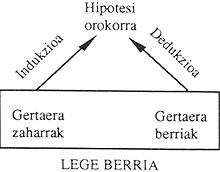The process leading to the scientific method is as follows:

Experimentally concrete facts are observed. These concrete observations are often expressed by the term singular enunciation.
When we have an appropriate set of observations and using the inductive method, we come to a general hypothesis.
Then, applying the deductive laws to this general hypothesis, new facts and the ancient facts we had before inducing are described.
1. But this process does not close in that cycle. With the passage of time a series of observations begins to appear that do not agree with the new law and that increase over time, both in quality and quantity. And in a historical moment the new law (or theory) we had before comes into crisis.

The response to this crisis generates a new theory. Therefore:

The laws obtained in the process of this open system are becoming wider (L 1 L 2 L 3 ..).

This is, in short, the operation of the scientific method.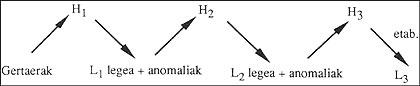## Newton's scientific method and method

Newton's theory has been a historically mentioned example to explain the functioning of the scientific method.

In the time of Newton some mechanical laws were already known (Kepler laws, free fall, sea tides, comet laws, etc. ), but there were no ties between them. Newton, through a process of abstraction (induction), created a general mechanics, laid the foundations of classical mechanics and on it the XVIII. and XIX. Over the centuries a very complex and mature building has been built.

In this construction Kant participated in giving a philosophical basis to Newton's physical system. Euler and Laplace fulfill the mathematics of the physical system with enormous power to Newton's apparatus. In this sense, it is worth highlighting the predictability of phenomena of classical mechanics. This competition became public with astronomy.

In 1846 the existence of another planet was postulated analyzing the anomalies of the planet Uranus in its orbits (using Newton's theory of gravitation). Astronomer Gottfried Galle discovered Neptune in a specific place in the Oratz, which later preached the theory. In this way, the empirical utility of Newton's theory was proven among all.

The Newtonian model will be a very complex system at maturity and will be called the kinetic-corpus system. Through this system appear all known phenomena in the universe at that time (except electromagnetic) defined according to the moving fractions.

But also in this system, so powerful and complete, there are beginning to emerge confictic observations. For example, the fluid that moves by the speed of light does not correspond to the values preached by classical mechanics, it is the mystery by which the orbits of electrons that exist in the atom and the radiation of the black body do not say. All these problems (and others) put classical mechanics into crisis until theories emerged that constitute the essence of current physics (Theory of relativity and quantum mechanics).

It seems, then, that in the case of Newton's theory the operation of scientific methodology is scrupulously carried out.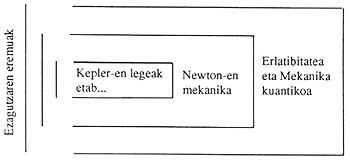## Science is jumping

Advertising According to the prestigious science philosopher Kuhn, scientific theories are constantly evolving. In this evolution there are advances, since the new theories cover more scientific problems than the old ones. But, and this is Kuhn's original criterion, these evolutions are not continuous, but discrete. If we look at the history of science, it is clear that scientific changes are making leaps and that the scientific process occurs through revolutions (and not by accumulation).

In this process jumping, in each leap there is a substitution of the paradigm (1), denying the old paradigm. Therefore, the new theory does not need the previous one, as the link of a process. No. The new theory breaks the old one. Old and new theory (paradigm) are incompatible; incompatible. Therefore, the scheme set forth in the first scientific method is not valid and should be interpreted as follows.

According to this scheme (Kuhn's) ancient theories provide nothing for the new ones. Each theory covers an increasingly broad field of knowledge, but there is no logical relationship between them.

According to this, the dynamics of Einstein and Newton are printers. If we accept Einstein's theory, this approval leads us to reject Newton's theory.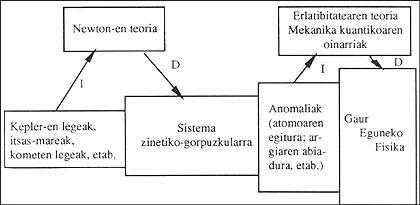## Unsurpassed chasm between Newton's and Einstein's mechanics

If certain conditions are established from relativistic mechanics (for example, if the relative speed between inertia systems is very low) it is possible to obtain Newtonian mechanics. Therefore, it seems that classical mechanics is a particular structure within relativity. And on such a level it is. Undoubtedly, classical and relativistic mechanics are related to measurement (the first is a particular case of the second). For a conventionalist and an instrumentalist the discussion ends here, because theories are only useful tools to dominate Nature.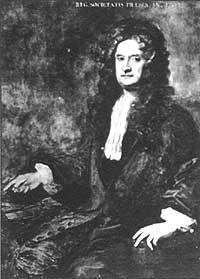Newton at 60.

But for the realist things are not like that. Scientific theories are tools for the realist.

But these instruments are not only for the domain of Nature. To understand nature. And according to this, concepts acquire greater interest than the measurements of a theory.

All this is very important when we analyze the theories of Newton and Einstein, since the concepts used by Newtonian mechanics (length, mass, etc.) are very different from those used by relativistic mechanics (relativistic length and relativistic mass). For example, in classical mechanical length it is defined by the wavelength of the spectrum, but a new element is introduced to define relativistic length, the relative speed between inertia systems. The same would happen with the dough.

Therefore, the physical significance of both theories is different, although in certain circumstances measurements are given in the same way.

But not only that. According to Kuhn and Feyerabend, these theories are not different, but exclusive. That is, if we use the concept of mass in Newtonian mechanics, this means that in relativity we cannot use the word mass, and if we do (and normally do) we are introducing a very dangerous mixture in the conceptualization of this theory. In this sense it can be said that some current scientists are making important efforts to redefine relativistic mass and relativistic length, using only the elements that appear in the theory.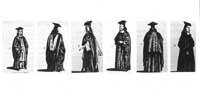Costumes in Cambridge XVII. In the 20th century. 3. Not graduated from Trinity College. 5 Backelor of Arts. 7, 8, 9 and 10 Masters of Arts.

From this path we can affirm the phrase that at first appeared as title: Between Newton's mechanics and Einstein's mechanics there is an insurmountable chasm.

## How to assess scientific theories?

To be consistent with what has been said so far, and as a conclusion, we affirm:

If Einstein's theory is true (and seems to exist at the moment), Newton's theory is false (even if it is applicable in concrete conditions).
When an alternative to relativity is created, relativity is said to be false and true is new. The only thing we can ask
of a theory is to give a coherent view of the universe according to its concepts. In this
historical process it can become a direction and theories considered false for a long time can be recovered. The long history of being wave/body of matter can be a clear example. Science,
therefore, is constantly changing by structuring increasingly broad and compressible theories, but we do not know (and do not know) whether they are true or false.
What each new theory does is give a new vision of reality.